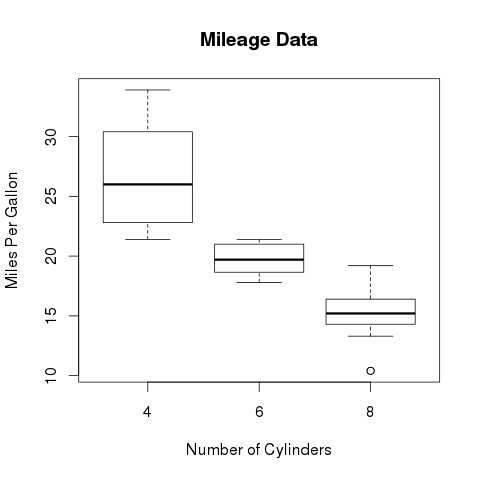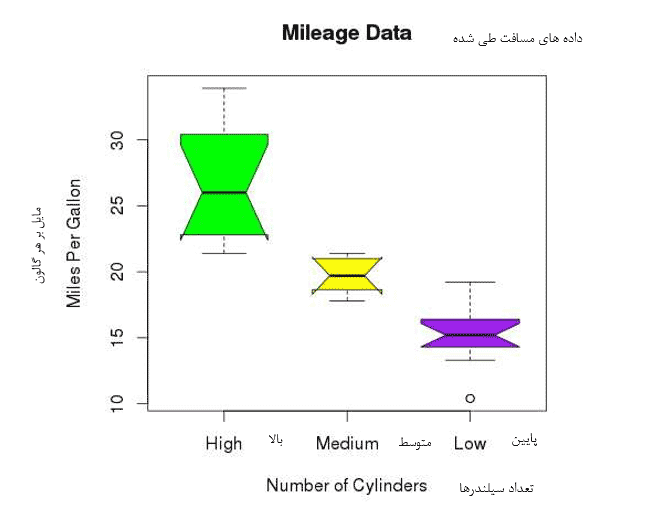# blog posts# Box charts; Is a measure of how data is distributed in a data set. Divides the data set into three to a quarter from the chart. This diagram shows the minimum, maximum, middle, first quadrant, and third quadrant in the data set, as well as a box diagram for each of them; It can be efficient to compare the distribution of data in a data set.

Box diagrams are created using the boxplot () function in R.

## Syntax

The basic syntax for creating a box diagram in R is as follows:

boxplot (x, data, notch, varwidth, names, main)

Parameters used in the above coding; as follows:

• x is a vector or a formula.
• data is a data framework.
• notch is a logical value. To draw depressions for each box; Must be set to TRUE.
• varwidth is a logical value. To draw the box width should be adjusted to fit the sample size.
• names A group of names that are printed under each box chart.
• main is used to present a title to the chart.

## Example

We used the “mtcars” dataset in the R environment to create a basic box diagram. Let’s take a look at the “mpg” and “cyl” columns in mtcars:

input <- mtcars [, c (‘mpg’, ‘cyl’)]

When we run the above code; The following result is obtain:

mpg cyl

Mazda RX4 21.0 6

Mazda RX4 Wag 21.0 6

Datsun 710 22.8 4

Hornet 4 Drive 21.4 6

Valiant 18.1 6

## Create box charts

The following script creates a box diagram for the relationship between mpg (miles per gallon) and cyl (number of cylinders).

# Give the chart file a name.

png (file = “boxplot.png”)

# Plot the chart.

boxplot (mpg ~ cyl, data = mtcars, xlab = “Number of Cylinders”,

ylab = “Miles Per Gallon”, main = “Mileage Data”)

# Save the file.

dev.off ()

When we run the above code; The following result is obtain:Miles per gallon

Number of cylinders

Distance data traveled

## Box diagram with recess

We can draw boxes with recesses to see how well the intermediates of different data sets match.

The following script creates a box chart with depressions for each data set.

# Give the chart file a name.

png (file = “boxplot_with_notch.png”)

# Plot the chart.

boxplot (mpg ~ cyl, data = mtcars,

xlab = “Number of Cylinders”,

ylab = “Miles Per Gallon”,

main = “Mileage Data”,

notch = TRUE,

varwidth = TRUE,

col = c (“green”, “yellow”, “purple”),

names = c (“High”, “Medium”, “Low”)

)

# Save the file.

dev.off ()

When we run the above code; We obtain the following Box diagrams result.